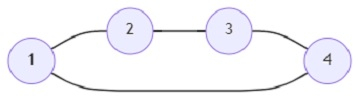# Program to find out the minimum size of the largest clique in a graph (Python)

Suppose we are given a graph and are asked to find out the minimum size of the largest clique in the graph. A clique of a graph is a subset of a graph where every pair of vertices are adjacent, i.e. there exists an edge between every pair of vertices. Finding the largest clique in a graph is not possible in polynomial time, so given the number of nodes and edges of a small graph we shall have to find out the largest clique in it.

So, if the input is like nodes = 4, edges =4; then the output will be 2.In the graph above, the maximum size of a clique is 2.

To solve this, we will follow these steps −

• Define a function helper() . This will take x, y
• ga := x mod y
• gb := y - ga
• sa := quotient of value of(x / y) + 1
• sb := quotient of value of(x / y)
• return ga * gb * sa * sb + ga *(ga - 1) * sa * sa / 2 + gb * (gb - 1) * sb * sb / 2
• i := 1
• j := nodes + 1
• while i + 1 < j, do
• p := i + floor value of((j - i) / 2)
• k := helper(nodes, p)
• if k < edges, then
• i := p
• otherwise,
• j := p
• return j

## Example

Let us see the following implementation to get better understanding −

import math
def helper(x, y):
ga = x % y
gb = y - ga
sa = x // y + 1
sb = x // y
return ga * gb * sa * sb + ga * (ga - 1) * sa * sa // 2 + gb * (gb - 1) * sb * sb // 2

def solve(nodes, edges):
i = 1
j = nodes + 1
while i + 1 < j:
p = i + (j - i) // 2
k = helper(nodes, p)
if k < edges:
i = p
else:
j = p
return j

print(solve(4, 4))


## Input

4,4

## Output

2

Updated on: 06-Oct-2021

112 Views